In the last post, we explored how a graph can be represented using an adjacency list. We’ll now look at how an adjacency matrix can also be used.

## Disclaimer

This post is mainly for me to jot down points about Adjacency Matrices. If this is helpful to you in some way, please let me know!

## What is an Adjacency Matrix?

An adjacency matrix is a square matrix representation of a finite graph. The elements indicate whether a pair of vertices is adjacent to each other.

A graph with `V` vertices can be represented with a `V x V` matrix (`V` rows and `V` columns) where:

• The row index represents the origin of the edge
• The column index represents the tail of the edge

Therefore, a point `(i, j)` represents an edge starting from `i` and ending at `j`.

If the value at this point `(i, j)` is:

• `1` then there is an edge from `i` to `j`
• `0` then there is no edge from `i` to `j`

We have previously looked at the following graph:In order to build this matrix, we would first need to create a `VxV` matrix full of zeros. `V` is the number of vertices which for this graph is 6 so we need to build a matrix consisting of 6 rows and 6 columns.

The `6x6` matrix looks like this: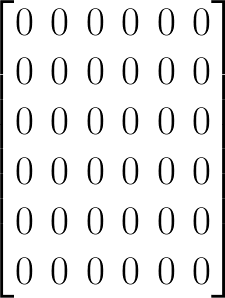The rows and columns are enumerated from 0 until 5 which corresponds with vertices `A` to `F` respectively.

We now go through each vertex and mark each node is connected to with a 1. Let’s start with vertex `A`.

### Vertex A

Vertex `A` is connected to both `B` and `C`.

1. Find the row corresponding to `A`: 0
2. Then we find the column for `B`: 1
3. Mark this point at row 0 and column 1 (`(0, 1)`) with a value of 1
4. Then we find the column for `C`: 2
5. Mark this point row 0 and column 2 (`(0, 1)`) with a value of 1

The resulting row for `A` now looks like: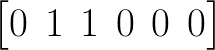### Vertex B

Vertex `B` is connected to both `D` and `E`.

1. Find the row corresponding to `B`: 1
2. Then we find the column for `D`: 3
3. Mark this point at row 1 and column 3 (`(1, 3)`) with a value of 1
4. Then we find the column for `E`: 4
5. Mark this point row 1 and column 4 (`(1, 4)`) with a value of 1

The resulting row for `B` now looks like: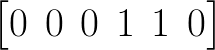### Vertex C

Vertex `C` is only connected to `F`.

1. Find the row corresponding to `C`: 2
2. Then we find the column for `F`: 5
3. Mark this point at row 2 and column 5 (`(2, 5)`) with a value of 1

The resulting row for `C` now looks like: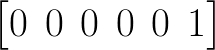### Vertex D, E, and F

Vertex `D`, `E`, and `F` have no adjacent nodes.

Therefore, their respective rows can remain as zeros.

## The Result

The completed adjacency matrix for the graph looks like: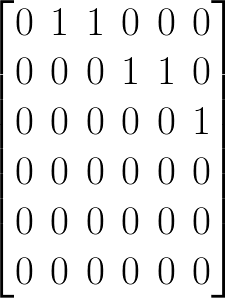Recall:

• `A` connects to both `B` and `C` so both `(0, 1)` and `(0, 2)` are marked with a `1`
• However, the point at `(1, 0)` is not marked with a point since `B` does not connect back to `A`.

## Implementation

The adjacent matrix can be implemented using a 2D array (aka an array of arrays).

• The inner arrays represent the columns
• The outer array represent the rows

The above matrix would be defined in Swift as follows:

``````let matrix: [[Int]] = [
[0, 1, 1, 0, 0, 0],
[0, 0, 0, 1, 1, 0],
[0, 0, 0, 0, 0, 1],
[0, 0, 0, 0, 0, 0],
[0, 0, 0, 0, 0, 0],
[0, 0, 0, 0, 0, 0]
]
``````

### Checking for Existence

If we wanted to determine whether there exists an edge between two vertices, we can do a lookup in this array to determine this. Recall that a value of 1 signifies the existence of an edge.

``````func hasEdge(in matrix: [[Int]], from row: Int, to col: Int) -> Bool {
// 1
guard isIndexValid((row, col), in: matrix) else { return false }
// 2
return matrix[row][col] == 1
}
``````
1. Calls a helper function that takes in a tuple of the row and column pair and returns if it’s valid for the given matrix
2. Accesses the value at the given row and column and verifies if it equals to 1
• This is sometimes easier to follow when separated:
``````  let rows = matrix[row]
return rows[col] == 1
``````

### Depth First Search (DFS)

When we did DFS on the graph, we did pre-order traversal that follows these steps:

1. Visit node
2. Visit left child
3. Visit right child

The result of the traversal was a visitation order as follows:

``````A -> B -> D -> E -> C -> F
``````

The DFS traversal for a matrix requires that we traverse as follows:

• Iterate through all the columns until we’ve found a `1` and if we haven’t visited it yet
• Given the column, repeat the DFS using this column as the new row
• Repeat these steps until all rows have been traversed
``````// This algorithm is courtesy of my colleague Michelle Lee!
func dfsMatrix(_ matrix: [[Int]], row: Int = 0) {
guard !matrix.isEmpty else { return }

print(edgesLookup[row])
// 1
rowsVisited.insert(row)

// 2
let colCount = matrix[row].count
for col in 0..<colCount {
// 3
if matrix[row][col] == 1 && !rowsVisited.contains(col) {
// 4
dfsMatrix(matrix, row: col)
}
}
}
``````
1. After printing the node that corresponds to the row, add this row to our set of visited nodes
2. Grab the count of columns for this given row and create a loop from `0` up to `colCount`
3. Check if the value at `(row, col)` is equal to `1` and that we haven’t visited this node before
4. Using this column `col`, repeat the DFS starting at this row.

The output should be the same as before:

``````A -> B -> D -> E -> C -> F
``````

This does assume that the first row has at least one edge through which for most cases is a valid assumption.

## Summary

An adjacency matrix is another way to represent a graph that makes lookups very quick.

However, there is often a lot of space required to define the graph. For large graphs, this can become quite inefficient.

A common example where you’ll see adjacency matrices is in this classic interview question: Island Problem. The example code can be found here.

Pros:

• The existence of an edge between two vertices can be determined in constant time: `O(1)`
• To determine if an edge exists can be done as: `matrix[i][j] == 1`

Cons:

• A `V * V` matrix is required even if only a few vertices have edges between them: `O(V^2)`
• Observe how the matrix above is mainly zeros
• Iterating through all the edges would exhaust the whole matrix in the worst case: `O(V^2)`
• If you need to iterate through all edges you would need to loop through the whole matrix